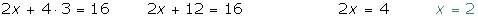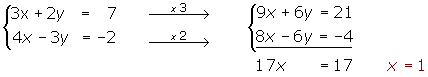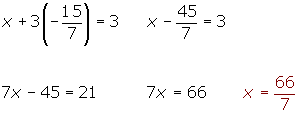Chapters

## 1

Prepare the two equations and multiply by the appropriate numbers in order to eliminate one of the unknown values.

## 2

Add the systems and eliminate one of the unknowns.

## 3

Solve the resulting equation.

## 4

Substitute the value obtained into one of the initial equations and then solve.

## 5

The two values obtained are the solution of the system.The easiest method is to remove the y, this way the equations do not have to be prepared. However, by choosing to remove the x, the process can be seen better.Replace the value of y in the second equation.Solution:Did you like the article?(1 votes, average: 5.00 out of 5)Loading...

Emma

I am passionate about travelling and currently live and work in Paris. I like to spend my time reading, gardening, running, learning languages and exploring new places.

Did you like
this resource?

Bravo!